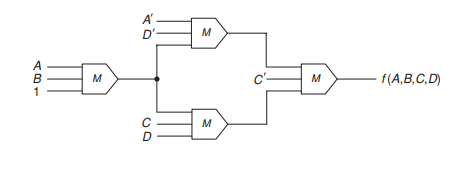# Digital-Grandtest1-Q2

The majority function is equal to 1 when two or three of its arguments equal 1, that is,What is the value ofasked Jun 19 in Digital
reshown Jun 20

+1 vote

Solution:

We have to find f(A,A,B,B) for given f(A,B,C,D)

Hence, replace B with A, C with B, D with B.Now for first M - The output is A. (i.e when A=1, Mout=1. when A=0, Mout=0)  Hence, A

For top M: For two inputs to be 1 B' has to be 1. Since one and always one of A , A' will be 1.

Similarly for bottom M: B has to be 1.  (for two inputs to be 1)

Hence , For final M, inputs are B',B' and B. For atleast two inputs to be 1. B' has to be 1.

Hence final f(A,A,B,B)=1, when B' =1.

Hence, Ans- B'answered Jun 21 by (83,420 points)
+1 vote
Here f(A,A,B,B) ASK so first convert all B to A and all C to B and all D to B

Now first level M gate input is AA1 so output is A.

In 2nd level first M gate input A'B'A

Output A'B'+0+AB'=B'(A+A')=B'

In 2nd level second M gate input is ABB

Output AB+AB+B=B

3rd level M gate input is B'BB'

Output is BB'+B'+BB'=0+B'+0=B'

Final output is B'

C is correct one.answered Jun 20 by (30,120 points)Option C is right oneanswered Jun 20 by (6,690 points)
edited Jun 20
Bro here B+B'+AB=1 not AB
So (B'+B').1.1=B'
SO C IS CORRECT
Oh yeah right
corrected... thanks
Welcome bro....